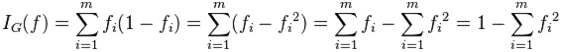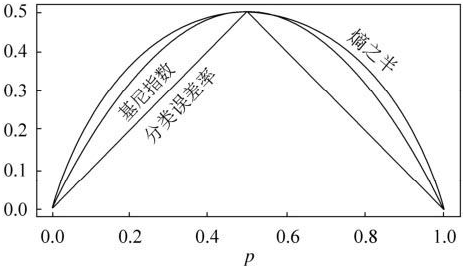# 决策树中基尼不纯度初步理解

一个随机事件变成它的对立事件的概率（简单理解）那么基尼不纯度就为   P(X=0)*(1 - P(X=0)) +  P(X=1)*(1 - P(X=1))  = 0.5

一个随机事件Y ，P(Y=0)= 0.1 ,P(Y=1)=0.9

那么基尼不纯度就为P(Y=0)*(1 - P(Y=0)) +   P(Y=1)*(1 -P(Y=1))  = 0.18

很明显 X比Y更混乱，因为两个都为0.5 很难判断哪个发生。而Y就确定得多，Y=1发生的概率很大。而基尼不纯度也就越小。

（1）基尼不纯度可以作为 衡量系统混乱程度的 标准；

（2）基尼不纯度越小，纯度越高，集合的有序程度越高，分类的效果越好；

（3）基尼不纯度为 0 时，表示集合类别一致；

（4）在决策树中，比较基尼不纯度的大小可以选择更好的决策条件（子节点）。

Python3示例代码：

#示例代码：
my_data = [['fan', 'C', 'yes', 32, 'None'],
['fang', 'U', 'yes', 23, 'Premium'],
['ming', 'F', 'no', 28, 'Basic']]

# 计算每一行数据的可能数量
def uniqueCounts(rows):
results = {}
for row in rows:
# 对最后一列的值计算
#r = row[len(row) - 1]
# 对倒数第三的值计算，也就是yes 和no 的一列
r = row[len(row) - 3]
if r not in results: results[r] = 0
results[r] += 1
return results

# 基尼不纯度样例
def giniImpurityExample(rows):
total = len(rows)
print(total)
counts = uniqueCounts(rows)
print(counts)
imp = 0
for k1 in counts:
p1 = float(counts[k1]) / total
print(counts[k1])
for k2 in counts:
if k1 == k2: continue
p2 = float(counts[k2]) / total
imp += p1 * p2
return imp

gini = giniImpurityExample(my_data)
print('gini Impurity is %s' % gini)